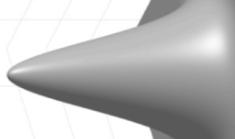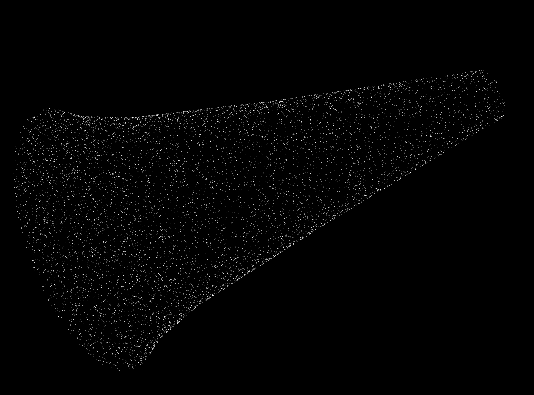# How to construct a gaussian geometry?

Dear experts,

Does anyone know how to construct a gaussian-like shaped detector geometry?

The length or thickness of the detector and the radius of the detector follow a gaussian function:

L = Gaus (R, mean = 0, sigma)

as shown in the figure:Best,
Yongke

You can probably approximate a gaussian using a connical geometery

https://geant4-userdoc.web.cern.ch/UsersGuides/ForApplicationDeveloper/html/Detector/Geometry/geomSolids.html

Otherwise, you can convert a CAD model into a tessellated surface and import into a G4TessellatedSolid

Hi,

An alternative to CAD or Tessellated solid is the `Polycon`, ie

``````    auto R0 = 1*cm;
auto zmax = 1*cm;
auto sigma=0.1*cm;
std::vector<G4double> zPlane = {0.05*zmax, 0.1*zmax, 0.2*zmax, 0.5*zmax, 0.9*zmax};
auto numZPlanes = zPlane.size();
std::vector<G4double> rInner( numZPlanes, 0.0 );
// function that calculates the outer radius as gausian.
// Takes by reference R0 and sigma
auto fgaus = [&](double x){return sigma*std::sqrt(-2*std::log(x/R0)); };
std::vector<G4double> rOuter = { fgaus(zPlane), fgaus(zPlane), fgaus(zPlane), fgaus(zPlane), fgaus(zPlane) };

G4Polycone*         targetSolid   = new G4Polycone("solid-Target",    // name
0.0,  		  // G4double  phiStart,
2*pi, 		  // G4double  phiTotal,
numZPlanes,	  // G4int     numZPlanes,
zPlane.data(),	  // const G4double  zPlane[],
rInner.data(),	  // const G4double  rInner[],
rOuter.data()	  // const G4double  rOuter[])
);
``````

Which results in:I hope it worksBest,
Alvaro

Dear Alvaro,

That sounds quite a good idea. Thanks very much!

Best,
Yongke

Dear loydms,